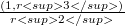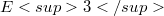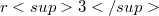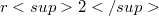﻿

### Newton\'s observations about the field of a uniform thin spherical shell

#### Abstract

Newton observed that with his law of gravitation the field outside a thin uniform spherical surface is the same as that of a particle at the center, having the same mass as the sphere.He also showed that the field is 0 inside a sphere. We find below all laws of central force having one or the other of these properties. We also formulate the analogous question in two and four (rather than just three) dimensions.We present the findings in a table where, for example, the entry$\frac{(1,r3)}{r2}$ in the place labelled «outside, 3» says that the fields that satisfy the condition outside every sphere in$E3$ are governed by laws which have any linear combination of 1 and$r3$ in the numerator, and$r2$ in the denominator.$$\begin{array}{ccc} \textrm{Dimension} & \textrm{Outside} & \textrm{Inside}\\ 2 & \frac{(1,r2)}{r} & \frac{(1,\log r)}{r} \\[5pt] 3 & \frac{(1,r3)}{r2} & \frac{(1,r2)}{r2} \\[5pt] 4 & \frac{(1,r2)}{r3} & \frac{(1,r)}{r3} \end{array}$$.

DOI Code: 10.1285/i15900932v10supn1p39

Full Text: PDF